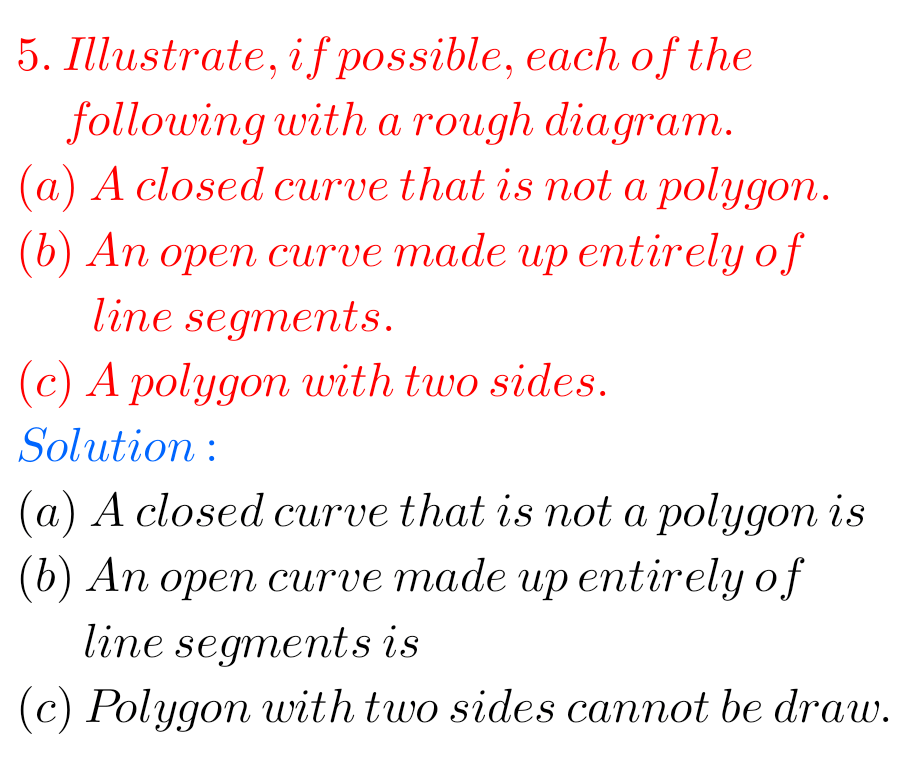## Maths Basic Geometrical Ideas Solutions exercise 4.2 class 6 Ncert

Basic Geometrical Ideas Solutions exercise 4.2 Class 6 Ncert Maths Ncert textbook Mathematics Class 6 Chapter 4 Basic Geometrical Ideas exercise 4.1 solutions are given. Study the lesson Basic Geometrical Ideas in the texbook. Observe the problems and solutions given in the text book. Observe the given below problems and solutions and try them in …